image1image2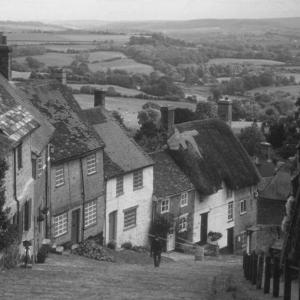function result = dim_image(img)
%将图像的每个像素点上的像素值均按下式进行变换
%x_n = 0.5*x_p^2
%提示：matlab对于double类型的图像，需要其绝对值不大于1才能进行显示。因此你需要进行归一化处理。
img=im2double(img);
[a,b,c]=size(img);
for k=1:c
for i=1:a
for j=1:b
img(i,j,k)=0.5*(img(i,j,k)^2);
end
end
end
result=img;
end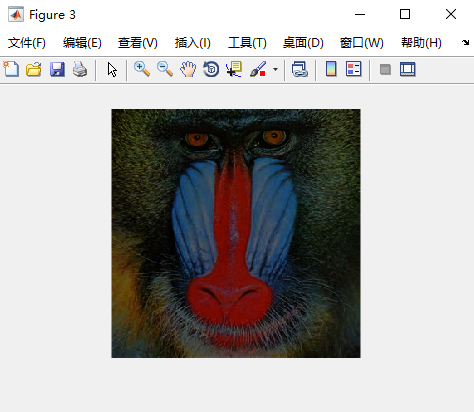function result=convert_to_grey_scale(img)
%本函数将彩色图像img转化成灰度图像result，转化公式如下：
%Gray = 0.299R + 0.587G + 0.114B
%提示：matlab对于double类型的图像，需要其绝对值不大于1才能进行显示。因此你需要进行归一化处理。
img=im2double(img);
[a,b,c]=size(img);
result=ones(a,b);
for k=1:c
for i=1:a
for j=1:b
result(i,j)=0.299*img(i,j,1)+0.587*img(i,j,2)+0.114*img(i,j,3);
end
end
end
end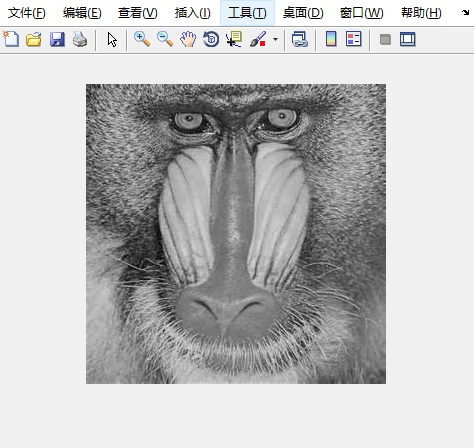function result=rgb_exclusion(img,channel)
%本函数从彩色图像img除去指定的通道channel
%对于被去除的通道，直接将该通道值置为0即可
result=img;
if channel=='R'
result(:,:,1)=0;
end
if channel=='G'
result(:,:,2)=0;
end
if channel=='B'
result(:,:,3)=0;
end

end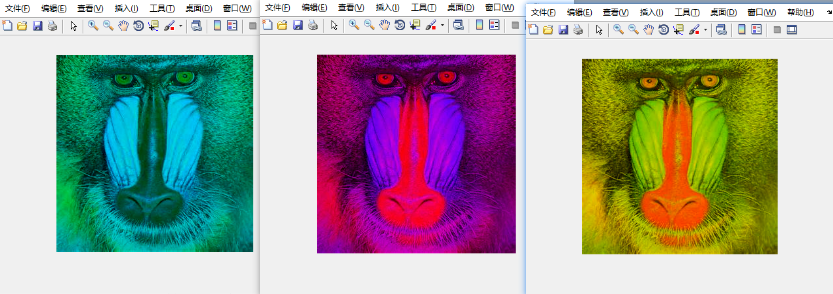function result=lab_decomposition(img,channel)
%本函数将输入图片分解为L,A,B三个通道
%并返回指定通道的图像
%提示：matlab对于double类型的图像，需要其绝对值不大于1才能进行显示。因此你需要进行归一化处理。
%提示：对于L通道，除以100即可；对于a通道，一般除以120即可；对于b通道，一般除以90即可
%提示：可以在命令行窗口键入help rgb2lab查看帮助
img=rgb2lab(img);
if channel=='L'
result=img(:,:,1)/100;
end
if channel=='A'
result=img(:,:,2)/120;
end
if channel=='B'
result=img(:,:,3)/90;
end

end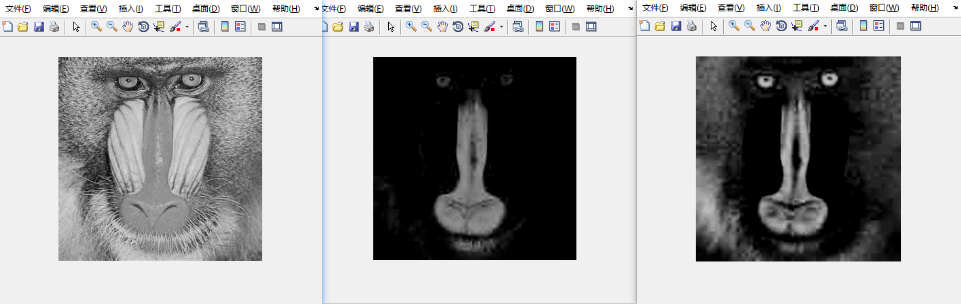function result=hsv_decomposition(img,channel)
%本函数将输入图片分解为H,S,V三个通道
%并返回指定通道的图像
%提示：matlab对于double类型的图像，需要其绝对值不大于1才能进行显示。因此你需要进行归一化处理。
%提示：可以在命令行窗口键入help rgb2hsv查看帮助
img=rgb2hsv(img);
if channel=='H'
result=img(:,:,1);
end
if channel=='S'
result=img(:,:,2);
end
if channel=='V'
result=img(:,:,3);
end
end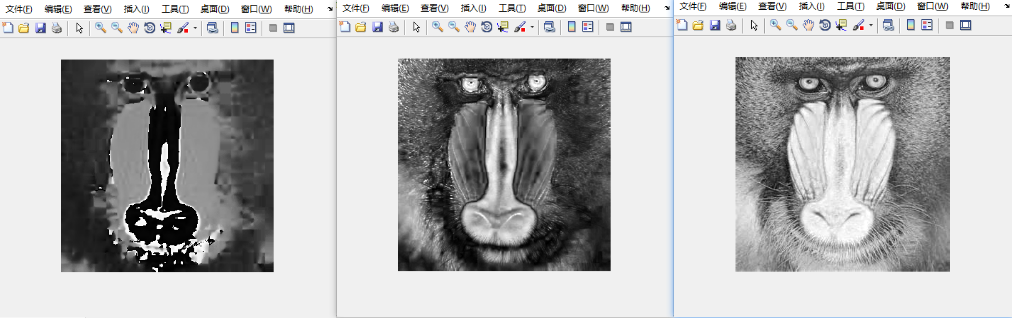function result=mix_images(img1,img2,channel1, channel2)
%本函数创建一幅新的图片，使得它的左半部分是图片1，右半部分是图片2，并且去掉指定的图像通道（R,G,或者B）。
[a,b,c]=size(img1);
img1=rgb_exclusion(img1,channel1);
img2=rgb_exclusion(img2,channel2);
for i=1:a
for j=b/2:b
img1(i,j,:)=img2(i,j,:);
end
end
result=img1;
end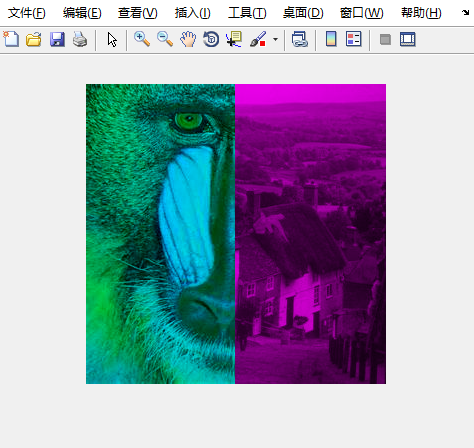mix_quadrants本函数将输入图像进行2*2的划分，即左上角四分之一，右上角四分之一，左下角四分之一以及右下角四分之一。对于每一个四分之一图像，都进行不同的操作（利用之前完成的函数）

function result=mix_quadrants(img1)
%本函数将输入图像进行2*2的划分，即左上角四分之一，右上角四分之一，左下角四分之一以及右下角四分之一。
%对于每一个四分之一图像，都进行不同的操作（利用之前完成的函数）：
%左上角四分之一：去除'R'通道
%右上角四分之一：变暗
%左下角四分之一：按该公式变亮：x_n = x_p^0.5
%右下角四分之一：去除'R'通道
%最终输出处理以后的图像
img1=im2double(img1);
[a,b,c]=size(img1);
result=img1;
im1=rgb_exclusion(img1,'R');
im2=dim_image(img1);
im3=img1;
for k=1:c
for i=1:a
for j=1:b
im3(i,j,k)=(im3(i,j,k)^0.5);
end
end
end
result(1:a/2,1:b/2,:)=im1(1:a/2,1:b/2,:);
result(a/2:a,1:b/2,:)=im3(a/2:a,1:b/2,:);
result(1:a/2,b/2:b,:)=im2(1:a/2,b/2:b,:);
result(a/2:a,b/2:b,:)=im1(a/2:a,b/2:b,:);

end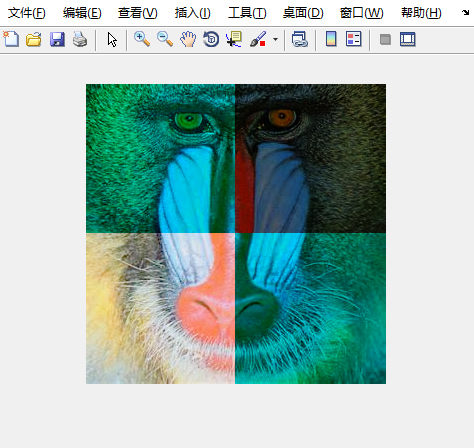Last modification：November 9th, 2020 at 09:55 pm